Special cases for laws of signsCustom SearchSPECIAL CASES Two special cases arise frequently in which the laws of signs may be used to advantage. The first such usage is in simplifying subtraction; the second is in changing the signs of the numerator and denominator when division is indicated in the form of a fraction. Subtraction The rules for subtraction may be simplified by use of the laws of signs, if each expression to be subtracted is considered as being multiplied by a negative sign. For example, 4 -(-5) is the same as 4 + 5, since minus times minus is plus. This result also establishes a basis for the rule governing removal of parentheses. The parentheses rule, as usually stated, is: Parentheses preceded by a minus sign may be removed, if the signs of all terms within the parentheses are changed. This is illustrated as follows: 12 -(3 - 2 + 4) = 12 - 3 + 2 - 4 The reason for the changes of sign is clear when the negative sign preceding the parentheses is considered to be a multiplier for the whole parenthetical expression. Division in Fractional Form Division is often indicated by writing the dividend as the -numerator, and the divisor as the denominator, of a fraction. In algebra, every fraction is considered to have three signs. The numerator has a sign, the denominator has a sign, and the fraction itself, taken as a whole, has a sign. In many cases, one or more of these signs will be positive, and thus will not be shown. For example, in the following fraction the sign of the numerator and the sign of the denominator are both positive (understood) and the sign of the fraction itself is negative: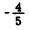Fractions with more than one negative sign are always reducible to a simpler form with at most one negative sign. For example, the sign of the numerator and the sign of the denominator may be both negative. We note that minus divided by minus gives the same result as plus divided by plus. Therefore, we may change to the less complicated form having plus signs (understood) for both numerator and denominator, as follows:Since - 15 divided by -5 is 3, and 15 divided by 5 is also 3, we conclude that the change of sign doss not alter the final answer. The same reasoning may be applied in the following ex- ample, in which the sign of the fraction itself is negative:When the fraction itself has a negative sign, as in this example, the fraction may be enclosed in parentheses temporarily, for the purpose of working with the numerator and denominator only. Then the sign of the fraction is applied separately to the result, as follows: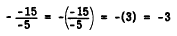All of this can be done mentally.  If a fraction has a negative sign in one of the three sign positions, this sign may be moved to another position. Such an adjustment is an ad- vantage in some types of complicated expressions involving fractions . Examples of this type of sign change follow:In the first expression of the foregoing example, the sign of the numerator is positive (understood) and the sign of the fraction is negative. Changing both of these signs, we obtain the second expression. To obtain the third expression from the second we change the sign of the numerator and the sign of the denominator. Observe that the sign changes in each case involve a pair of signs. This leads to the law of signs for �fractions: Any two of the three signs of a fraction may be changed without altering the value of the fraction. AXIOMS AND LAWS An axiom is a self-evident truth.. It is a truth that is so universally accepted that it does not require proof. For example, the statement that "a straight line is the shortest distance between two points" is an axiom from plane geometry. One tends to accept the truth of an axiom without proof, because anything which is axiomatic is, by its very nature, obviously true. On the other hand, a law (in the mathematical sense) is the result of defining certain quantities and relationships and then developing logical conclusions from the definitions. AXIOMS OF EQUALITY The four axioms of equality with which we are concerned in arithmetic and algebra are stated as follows: 1. If the same quantity is added to each of two equal quantities, the resulting quantities are equal. This is sometimes stated as follows: H equals are added to equals, the results are equal. For example, by adding the same quantity (3) to both sides of the following equation, we obtain two sums which are equal: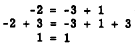2. If the same quantity is subtracted from each of two equal quantities, the resulting quantities are equal. This is sometimes stated as follows: If equals are subtracted from equals, the results are equal. For example, by subtracting 2 from both sides of the following equation we obtain results which are equal: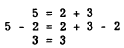3. If two equal quantities are multiplied by the same quantity, the resulting products are equal. This is sometimes stated as follows: If equals are multiplied by equals, the products are equal. For example, both sides of the following equation are multiplied by -3 and equal results are obtained: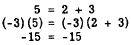4. If two equal quantities are divided by the same quantity, the resulting quotients are equal. This is sometimes stated as follows:. If equals are divided by equals, the results are equal. For example, both sides of the following equation are divided by 3, and the resulting quotients are equal:These axioms are especially useful when letters are used to represent numbers. If we know that 5x = -30, for instance, then dividing both 5x and -30 by 5 leads to the conclusion that x = -6. LAWS FOR COMBINING NUMBERS Numbers are combined in accordance with the following basic laws: 1. The associative laws of addition and multiplication. 2. The commutative laws of addition and multiplication. 3. The distributive law. Associative Law of Addition The word "associative" suggests association or grouping. This law states that the sum of three or more addends is the same regardless of the manner in which they are grouped. For example, 6 + 3 + 1 is the same as 6 + (3 + 1) or (6 +3) +1. This law can be applied to subtraction by changing signs in such a way that all negative signs are treated as number signs rather than operational signs. That is, some of the addends can be negative numbers. For example, 6 - 4 - 2 can be rewritten as 6 + (-4) + (-2). By the associative law, this is the same as     6 + [(-4) + (-2)] or [6 + (-4)] + (-2). However, 6 - 4 - 2 is not the same as 6 - (4 - 2); the terms must be expressed as addends before applying the associative law of addition. Associative Law of Multiplication This law states that the product of three or more factors is the same regardless of the manner in which they are grouped. For example, 6 x 3 x 2 is the same as (6 x  3) x 2 or 6 x (3 x 2). Negative signs require no special treatment in the application of this law. For exam le 6 - (-4) - (-2) is the same as [6 -(-4)].- (2) or 6 - [(-4) - (-2)]. Commutative Law of Addition The word "commute" means to change, substitute or move from place to place. The commutative law of addition states that the sum of two or more addends is the same regardless of the order in which they are arranged. For ex- ample, 4 + 3 + 2 is the same as 4 + 2 + 3 or 2+4+3. This law can be applied to subtraction by changing signs so that all negative signs become number signs and all signs of operation are positive. For example, 5 - 3 - 2 is changed to 5 + (-3) + (-2), which is the same as 5 + (-2) + (-3) or (-3) + 5 + (-2). Commutative Law of Multiplication This law states that the product of two or more factors is the same regardless of the order in which the factors are arranged. For example, 3 x 4 x 5 is the same as 5 x 3 x 4 or 4 x 3 x 5. Negative signs require no special treatment in the application of this law. For example, 2 * (-4) * (-3) is the same as (-4) x (-3) x 2 or (-3) x 2 x (-4). Distributive Law . This law .combines the operations of addition and multiplication. The word "distributive" refers to the distribution of a common multiplier among the terms of an additive expression. For example,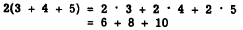To verify the distributive law, we note that 2(3 + 4 + 5) is the same as 2(12) or 24. Also, 6 + 8 + 10 is 24. For application of the distributive law where negative signs appear, the following procedure is recommended: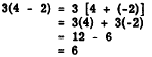Integrated Publishing, Inc. - A (SDVOSB) Service Disabled Veteran Owned Small Business